# 基于STM32的单相在线式不间断电源系统STM32-Based Single-Phase Online Uninterruptible Power Supply System

DOI: 10.12677/SEA.2020.96053, PDF, HTML, XML, 下载: 139  浏览: 439

Abstract: This design is based on STM32 single-chip microcomputer, using PWM modulation and SPWM modulation technology to control the two-way DCDC and single-phase inverter circuit respectively, and the overall hardware circuit is closed loop adjusted by PID algorithm, so as to realize the output of fixed frequency and Stable voltage UPS power system. This system mainly includes transformer module, two-way DCDC module, single-phase inverter module, AD637 AC sampling module. The UPS realizes the function of outputting sinusoidal alternating current with a fixed frequency and amplitude.

1. 引言

UPS电源系统是一种电源储能设备，可以不断地向一些关键设备(铁路和民航的售票系统、互联网数据中心、医院的生命维护设备、银行的清算中心等)提供频率稳定、精度高、失真度小的高质量正弦波 。然而传统的逆变电源多为模拟控制，虽然模拟控制技术已经非常成熟，但模拟电路存在电路复杂，灵活度不够，调试困难，产品升级慢等缺点。伴随着高速单片机和数字信号处理器的出现，使逆变电源的数字化控制成为现实，数字化控制也成为了UPS未来发展的一大趋势 。而本设计以直流电源代替蓄电池为前提条件，设计出一款可以稳定输出频率50 Hz，幅值有效值为30 V的UPS电源系统。

2. UPS不间断电源系统的总体设计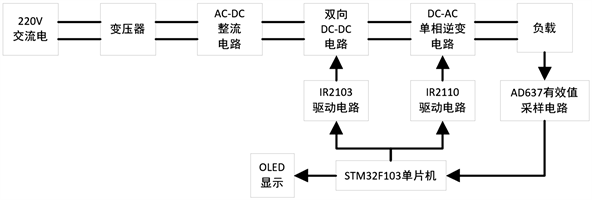Figure 1. Overall system framework

3. UPS不间断电源模块设计与选择

3.1. 主控单片机的选用

3.2. AC-DC整流模块的选择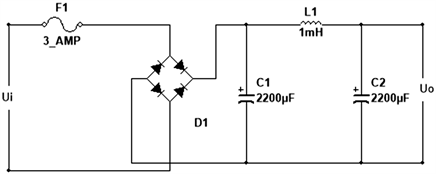Figure 2. AC-DC rectifier circuit diagram

3.3. 驱动电路设计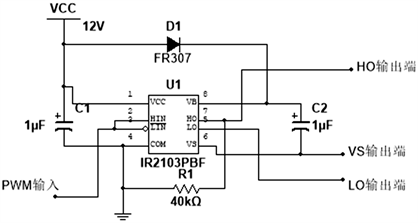Figure 3. IR2103 drive circuit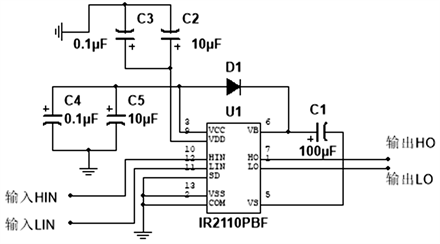Figure 4. IR2110 drive circuit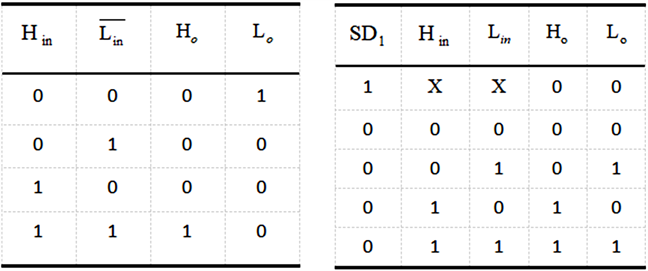Figure 5. IR2103 (left picture), IR2110 pin level output logic relationship

3.4. 升压模块的设计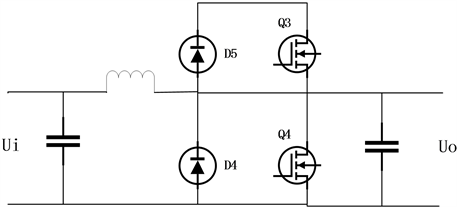Figure 6. Bidirectional DC-DC main circuit structure

$D=1-\frac{{V}_{in}}{{V}_{o}}=\frac{60-43}{60}=0.283$ (1)

${I}_{av}=\frac{{P}_{o}}{\eta \cdot {U}_{i\left(\mathrm{min}\right)}}=\frac{60×1}{0.95×29}=2.178\text{\hspace{0.17em}}\text{A}$ (2)

$\Delta I=0.2{I}_{av}=0.2×2.178=0.4356\text{\hspace{0.17em}}\text{A}$ (3)

${L}_{boost}=\frac{\left({U}_{out}-{U}_{in}\right)\cdot \left(1-D\right)}{\Delta I\cdot f}=349\text{\hspace{0.17em}}\mu \text{H}$ (4)

$\Delta Q=\Delta I\cdot \frac{T}{2}=\Delta I\cdot \frac{1}{2f}=2.723\text{\hspace{0.17em}}\mu \text{C}$ (5)

${C}_{boost}\ge \frac{\Delta Q}{\Delta U}=\frac{2.723\text{\hspace{0.17em}}\mu \text{C}}{0.01\text{\hspace{0.17em}}\text{V}}=272.3\text{\hspace{0.17em}}\mu \text{F}$ (6)

3.5. 单相逆变模块的设计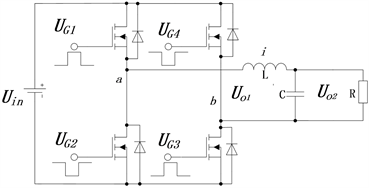Figure 7. Single-phase inverter circuit

3.6. 交流采样模块的设计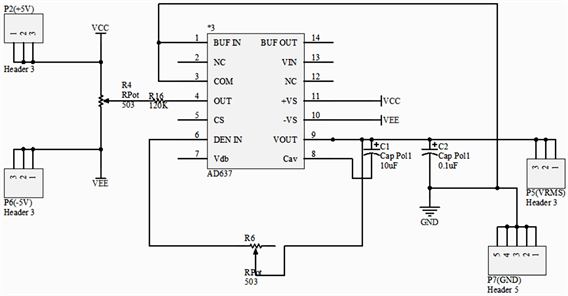Figure 8. AD637 effective value detection circuit

1) 无论真有效值的输出波形复杂程度如何都能计算, 还可以输出分贝值。

2) 频带宽，量程可调；当输入Uin = 200 mV时，上限频率为600 kHz，当Uin大于1 V时，上限频率可达8 MHz；

3) 常情况下不需要外部元件，唯一的外接元件是平均电容Cav，当接上Cav时，构成低通滤波器, 只要滤波时间常数RC远远大于输入信号的周期，输出信号值就是任意输入信号的真有效值，若不接平均电容Cav，AD637就进行绝对值计算。

4) AD637芯片内部有独立的缓冲放大器，可作为输入缓冲器来用，还可构成有源滤波器来减小纹波，提高测量精度。

5) 输出端有过压保护电路，当输入超过电源电压，基本不会烧坏器件，所以不需要限幅电路来保护，并且电源电压宽，为±3 V~±18 V 。

4. UPS不间断电源系统的软件设计

4.1. 软件整体设计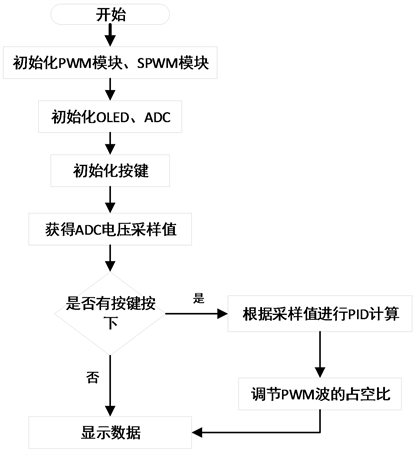Figure 9. The overall framework of software design

4.2. 稳压控制算法实现

$u\left(t\right)={K}_{p}\left(e\left(t\right)+\frac{1}{{T}_{t}}{\int }_{0}^{t}e\left(t\right)\text{d}t+{T}_{D}\frac{\text{d}e\left(t\right)}{\text{d}t}\right)$ (7)

Kp表示比例增益，Tt表示积分时间常数，TD表示微分时间常数，u(t)表示输出的信号，e(t)表示采样值和基准值的偏差。而在软件的实现中，采用队列的思想，读取10个值差值，去掉最原始的差值，保留最新的差值，根据公式计算出差的值即可，具体的流程图如图10所示。

4.3. PWM和SPWM调制技术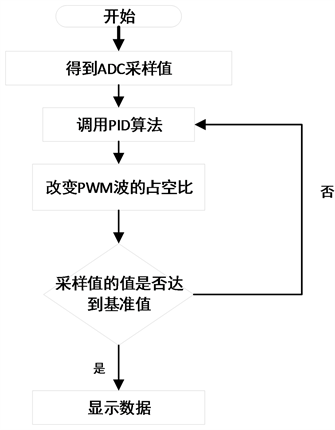Figure 10. Flow chart of voltage stabilization algorithm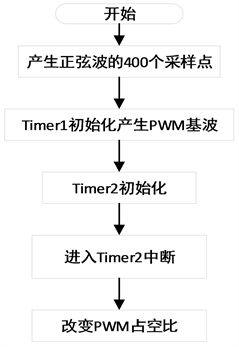Figure 11. SPWM wave generation

if(i<201)

{

TIM_SetCompare1(TIM1,(u16)(Period_percent*spwm1[i++])); //修改TIM1通道1的PWM占空比，后者为捕获/比较寄存器1的值

}

else if(i>200&&i<400)//一周期采样400个点

{

TIM_SetCompare1(TIM1,0);

i++;

}

else if(i== 400)

i = 0;

if(j<400)//一周期采样400个点

TIM_SetCompare3(TIM1,(u16)(Period_percent*spwm2[j++]));

else if(j== 400)

j = 0 ;

}

4.4. ADC采样

void Get_Adc_Average2_init(u8 ch)

{

for (i=0;i<10;i++)

{

value[i]=Get_Adc2(ch);

temp_val1=temp_val1+value[i];

}

}

u16 Get_Adc_Average2(u8 ch)

{

if(i==10)i=0;

temp_val1=temp_val1-value[i];

value[i]=Get_Adc2(ch);

temp_val1=temp_val1+value[i];

i++;

return temp_val1/10;

delay_ms(5);

}

5. 测试方案与结果

1) 测试Ui = 36 V, Io = 1 A时输出电压Uo和频率f，结果见表1Table 1. Record of output voltage and frequency under AC power supply

2) 通过调整负载，输出电流Io为0.1 A、1 A时对应的输出电压Uo，结果见表2Table 2. Adjust the load output current and voltage record

3) 测试负载调整率，Ui为43 V、29 V时对应的输出电压Uo，结果见表3Table 3. Adjust voltage output current and voltage record

4) 断开交流电，即时切换至直流(储能器件侧)供电，Ud = 24 V，输出交流电流Io = 1 A时，输出交流电压Uo = 30 V ± 0.2 V，频率为f = 50 Hz ± 0.2 Hz，结果见表4Table 4. DC power supply inverter voltage record

5) 硬件实物图如图12所示。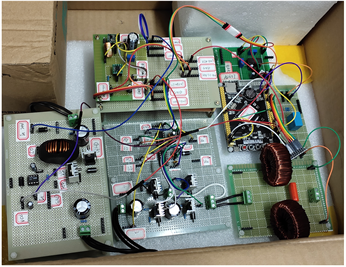Figure 12. Physical picture of the overall circuit

6. 结论

NOTES

*第一作者。

#通讯作者。

  唐剑青. 数字化不间断电源的研究[D]: [硕士学位论文]. 杭州: 浙江大学, 2006.  李伟. 数字控制逆变电源的研究与实现[D]: [硕士学位论文]. 武汉: 武汉理工大学, 2007.  张凌雪. 基于STM32F103的智能小车设计[J]. 农家参谋, 2020(23): 106.  厉俊, 郑佳蕙. 基于STM32的开关电源模块并联供电系统[J]. 软件工程, 2020, 23(3): 4-8.  刘波粒, 刘彩霞. 模拟电子技术基础[M]. 北京: 高等教育出版社, 2016.  申浩锋, 高宁波. 基于IR2103的步进电机全桥驱动电路设计[J]. 微电机, 2016, 49(10): 67-71.  贺根华, 祁承超, 柳鑫. 基于DSP28335 + IR2110芯片的移相全桥驱动电路设计[J]. 惠州学院学报, 2018, 38(3): 67-70.  于玉军, 王亚君, 陈垚. 小型高效数字式双向DC/DC变换器设计[J]. 电子器件, 2020, 43(5): 1029-1034.  程星, 黄要然, 艾皓, 谢作生. 基于tms320f28027的逆变电源制作[J]. 电子制作, 2019(1): 12-14+5.  余海宁, 邵红艳, 邱望标. 非正弦波中频强磁场测量方法及装置[J]. 机械与电子, 2017, 35(8): 45-49+53.  崔建伟. 基于嵌入式系统的数字滤波器、倾角传感器设计[D]: [硕士学位论文]. 北京: 北京邮电大学, 2008.